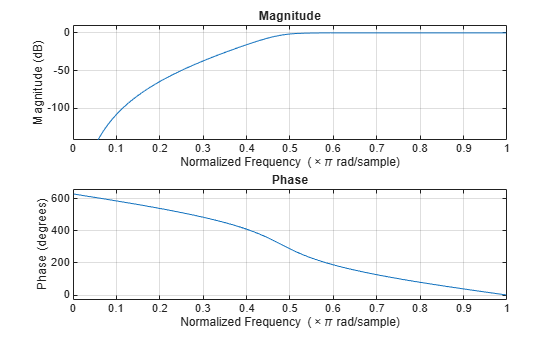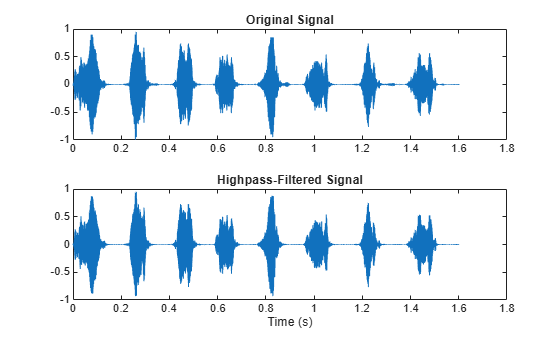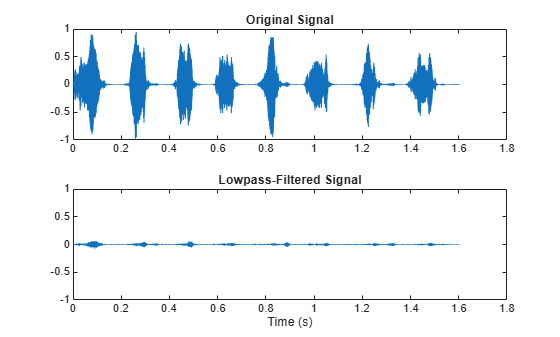Documentation

# sosfilt

Second-order (biquadratic) IIR digital filtering

## Syntax

```y = sosfilt(sos,x) y = sosfilt(sos,x,dim) ```

## Description

`y = sosfilt(sos,x)` applies the second-order section digital filter `sos` to the vector `x`. The output, `y`, is the same length as `x`.

### Note

If either input to `sosfilt` is single precision, filtering is implemented using single-precision arithmetic. The output, `y`, is single precision.

`sos` represents the second-order section digital filter H(z)

`$H\left(z\right)=\prod _{k=1}^{L}{H}_{k}\left(z\right)=\prod _{k=1}^{L}\frac{{b}_{0k}+{b}_{1k}{z}^{-1}+{b}_{2k}{z}^{-2}}{1+{a}_{1k}{z}^{-1}+{a}_{2k}{z}^{-2}}$`

by an L-by-6 matrix containing the coefficients of each second-order section in its rows.

`$\text{sos}=\left[\begin{array}{cccccc}{b}_{01}& {b}_{11}& {b}_{21}& 1& {a}_{11}& {a}_{21}\\ {b}_{02}& {b}_{12}& {b}_{22}& 1& {a}_{12}& {a}_{22}\\ ⋮& ⋮& ⋮& ⋮& ⋮& ⋮\\ {b}_{0L}& {b}_{1L}& {b}_{2L}& 1& {a}_{1L}& {a}_{2L}\end{array}\right]$`

If `x` is a matrix, `sosfilt` applies the filter to each column of `x` independently. The output `y` is a matrix of the same size, containing the filtered data corresponding to each column of `x`.

If `x` is a multidimensional array, `sosfilt` filters along the first nonsingleton dimension. The output `y` is a multidimensional array of the same size as `x`, containing the filtered data corresponding to each row and column of `x`.

The second order sections matrix, `sos`, the input signal, `x`, or both can be double or single precision. If at least one input is single precision, filtering is done with single precision arithmetic.

`y = sosfilt(sos,x,dim)` operates along the dimension `dim`.

## Examples

collapse all

Load `chirp.mat`. The file contains a signal, `y`, that has most of its power above `Fs/4`, or half the Nyquist frequency. The sample rate is 8192 Hz.

```load chirp t = (0:length(y)-1)/Fs; ```

Design a 7th-order Butterworth highpass filter to attenuate the components of the signal below `Fs/4`. Use a normalized cutoff frequency of 0.48π rad/sample. Express the filter coefficients in terms of second-order sections.

```[zhi,phi,khi] = butter(7,0.48,'high'); soshi = zp2sos(zhi,phi,khi); freqz(soshi) ```Filter the signal. Display the original and highpass-filtered signals. Use the same y-axis scale for both plots.

```outhi = sosfilt(soshi,y); subplot(2,1,1) plot(t,y) title('Original Signal') ys = ylim; subplot(2,1,2) plot(t,outhi) title('Highpass-Filtered Signal') xlabel('Time (s)') ylim(ys) ```Design a lowpass filter with the same specifications. Filter the signal and compare the result to the original. Use the same y-axis scale for both plots. The result is mostly noise.

```[zlo,plo,klo] = butter(7,0.48); soslo = zp2sos(zlo,plo,klo); outlo = sosfilt(soslo,y); subplot(2,1,1) plot(t,y) title('Original Signal') ys = ylim; subplot(2,1,2) plot(t,outlo) title('Lowpass-Filtered Signal') xlabel('Time (s)') ylim(ys) ```## References

 Orfanidis, S.J., Introduction to Signal Processing, Prentice-Hall, Englewood Cliffs, NJ, 1996.